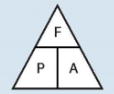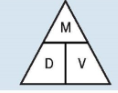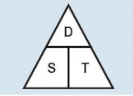# Compound Units

## Compound units 1

Compound measures are measures that require more than one unit. You will more than likely have come across these in science. You need to learn them off by heart and also how to rearrange them.Pressure (Newtons per unit2) =Force ( Newtons) / Area (units squared)Density (kg or g or mg/ units cubed)= Mass ( kg/g/mg) / Volume ( units cubed)Average Speed (units/time)= Distance(metres/miles/ units etc.) / Time ( mins/secs/hours etc.)

1. You can use the triangles to help you rearrange the formulas. Cover over the measure that you are trying to find, if the measure is at the top of the triangle, multiply the two bottom units. If the measure is on the bottom of the triangle divide the top unit by the other remaining unit.

Eg. If a car travels a distance of 10 miles and goes 30 miles per hour, how much time will it take to get to its destination?

As we are looking for the time we need to divide the distance by the speed__T__ime, the __S__peed= 30 miles per hour and the __D__istance=10 miles

Therefore 10/30= ⅓ of an hour.

1. This is where we have to be careful with our answer!

⅓ of an hour = 1/3rd of 60 minutes. So if they want the answer in hours keep it as ⅓ hour, if they want it in minutes it will be the answer is 20 minutes.

Rates of pay

Rates of pay are also compound measures. For example, if you earn £5 per hour, that uses two measures, money and time.

If the pressure is 50 newtons per m2 and the area is 20 metres, what is the force applied?
Your answer should include: 1000N / 1000 newtons / 1000
In the mass of an object is 120 g and it’s density is 5 g/cm3 , what is its volume?
Your answer should include: 24cm3 / 24cm^3 / 24
It takes a car 30 minutes to travel 5 miles, what is its average speed? (Give you answer in miles per hour)
Your answer should include: 10 / 10mph / 10m/h